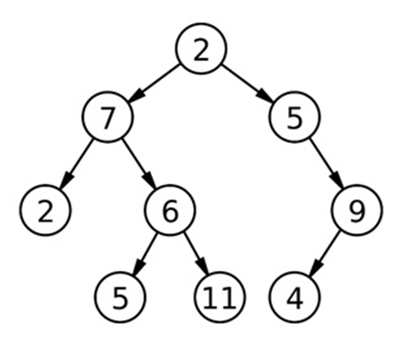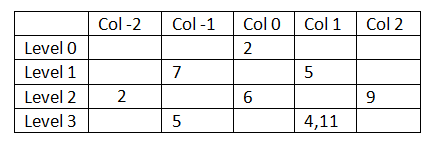# Print vertical sum of a binary tree

In this article, we are going to learn what vertical sum of a binary tree is and how to find it through a C++ program?
Submitted by Radib Kar, on November 30, 2018

Problem statement: Given a binary tree, find the vertical sum for the binary tree along each vertical line.

Solution:

First we need to understand what vertical sum is. Let go through an example to understand what vertical sum is.For the above tree, let's check what the vertical sum is for the tree.Just consider, we have partitioned the tree nodes into column & rows where rows are the level no starting from 0. Then the above tree can be converted into the above table easily.

1. Start from root. Root column is 0.
2. If next node is at left of current node
Then column of next node=column of current node-1
Else
Column of next node=column of current node+1

Using the above steps the tree can be easily partitioned to the table. It is to observe that there may be several entry at a specific (row, column) position. Like here, at Level3, column no 1 has two entry 4 & 11.

Rest is about doing sums for each column and printing it.

Thus the vertical sum output should be 2, 12, 8, 20, 9 (from col -2 to col 2 direction).

Though the visual description seems to be very easy to solve this problem, in programing view it’s not that easy.

## Algorithm to find vertical sum

The basic concept is to do pre-order traversal & while traversing we will keep track for each column (hashing)& will find cumulative sum.

Thus the column is used as key & we need a map to process our algorithm.

1. Initialize map<int, int>hash. //(ordered map)
2. Start from root. Column for root is 0.
Call vertical_sum(root, 0, hash);
3. Recursive function
4. ```Function vertical_sum( tree node, column, reference of map hash)
a) If (node== NULL)
Return;
//hash[column]+=node->data
b) Add node value cumulatively for the column;
// vertical_sum(node->left, column-1, reference of map hash)
c) Recursive do for the left subtree;
We are passing col-1 since it’s on the left
// vertical_sum(node->right, column+1, reference of map hash)
d) Recursive do for the right subtree;
We are passing col+1 since it’s on the right

End Functionvertical_sum;
```
5. Print the hash map to output the vertical sums for corresponding column.

## C++ implementation to print vertical sum of a binary tree

```#include <bits/stdc++.h>
using namespace std;

// tree node is defined
class tree{
public:
int data;
tree *left;
tree *right;
};

//finding vertical sum
void findVerticalSum(tree* root,int col,map<int,int> &hash){
if(root==NULL) //base case
return;
//finding sum for respecting column, hashing the column
hash[col]+=root->data;
//recursively process left sub-tree
findVerticalSum(root->left,col-1,hash);
//recursively process right sub-tree
findVerticalSum(root->right,col+1,hash);
}

void vertical_sum(tree* root){
//ordered hash map
map<int,int> hash;
//column no for root is 0
findVerticalSum(root,0,hash);
cout<<"column"<<"\t"<<"sum\n";
//printing the values from hash map
for(auto it=hash.begin();it!=hash.end();it++){
//it->first= column no(key) ,
//it->second=vertical sum of respective column(value)
cout<<it->first<<"\t"<<it->second<<endl;
}
}

// creating new node
tree* newnode(int data)
{
tree* node = (tree*)malloc(sizeof(tree));
node->data = data;
node->left = NULL;
node->right = NULL;
return(node);
}

int main()
{
//**same tree is builted as shown in example**
int c,K;
cout<<"Tree is built like the example aforesaid"<<endl;
//building the tree like as in the example
tree *root=newnode(2);
root->left= newnode(7);
root->right= newnode(5);
root->right->right=newnode(9);
root->right->right->left=newnode(4);
root->left->left=newnode(2);
root->left->right=newnode(6);
root->left->right->left=newnode(5);
root->left->right->right=newnode(11);

cout<<"finding vertical sums......"<<endl;
vertical_sum(root);

return 0;
}
```

Output

```Tree is built like the example aforesaid
finding vertical sums......
column  sum
-2      2
-1      12
0       8
1       20
2       9
```

Languages: » C » C++ » C++ STL » Java » Data Structure » C#.Net » Android » Kotlin » SQL
Web Technologies: » PHP » Python » JavaScript » CSS » Ajax » Node.js » Web programming/HTML
Solved programs: » C » C++ » DS » Java » C#
Aptitude que. & ans.: » C » C++ » Java » DBMS
Interview que. & ans.: » C » Embedded C » Java » SEO » HR
CS Subjects: » CS Basics » O.S. » Networks » DBMS » Embedded Systems » Cloud Computing
» Machine learning » CS Organizations » Linux » DOS
More: » Articles » Puzzles » News/Updates